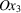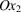International
Tables for
Crystallography
Volume D
Physical properties of crystals
Edited by A. Authier

International Tables for Crystallography (2006). Vol. D, ch. 1.1, p. 18

## Section 1.1.4.8.2. Monoclinic system

A. Authiera*

aInstitut de Minéralogie et de la Physique des Milieux Condensés, Bâtiment 7, 140 rue de Lourmel, 75015 Paris, France
Correspondence e-mail: aauthier@wanadoo.fr

| top | pdf |

#### 1.1.4.8.2.1. Group| top | pdf |

Choosing the twofold axis parallel toand applying the direct inspection method, one findsThere are 13 independent components. If the twofold axis is parallel to, one finds#### 1.1.4.8.2.2. Group m

| top | pdf |

One obtains the matrix representing the operator m by multiplying by −1 the coefficients of the matrix representing a twofold axis. The result of the reduction will then be exactly complementary: the components of the tensor which include an odd number of 3's are now equal to zero. One writes the result as follows:There are 14 independent components. If the mirror axis is normal to, one finds#### 1.1.4.8.2.3. Group| top | pdf |

All the components are equal to zero.